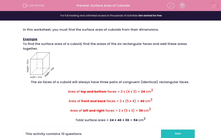# Surface Area of Cuboids

In this worksheet, students calculate the surface area of cuboids given their dimensions.This content is premium and exclusive to EdPlace subscribers.Key stage:  KS 2

Curriculum topic:   Maths and Numerical Reasoning

Curriculum subtopic:   3D Shapes

Difficulty level:#### Worksheet Overview

In this worksheet, you must find the surface area of cuboids from their dimensions.

Example

To find the surface area of a cuboid, find the areas of the six rectangular faces and add these areas together.The six faces of a cuboid will always have three pairs of congruent (identical) rectangular faces.

Area of top and bottom faces = 2 x (4 x 3) = 24 cm2

Area of front and back faces = 2 x (5 x 4) = 40 cm2

Area of left and right faces = 2 x (5 x 3) = 30 cm2

Total surface area = 24 + 40 + 30 = 94 cm2

### What is EdPlace?

We're your National Curriculum aligned online education content provider helping each child succeed in English, maths and science from year 1 to GCSE. With an EdPlace account you’ll be able to track and measure progress, helping each child achieve their best. We build confidence and attainment by personalising each child’s learning at a level that suits them.

Get started